## Friday, 22 June 2012

### CBSE Class 9 (Maths) - Polynomials - Exercise 2.4

Factor Theorem

1. Let p(x) be a polynomial and a be any real number. If p(a) = 0, then (x–a) is a factor of p(x).

2. If (x–a) is a factor of p(x), then p(a) = 0

EXERCISE 2.4 (NCERT Solution)

Q1: Determine which of the following polynomials has (x + 1) a factor :
(i) x3 + x2 + x + 1
(ii) x4 + x3 + x2 + x + 1
(iii) x4 + 3x3 + 3x2 + x + 1
(iv) x3 – x2 – (2 + √2)x + √2

Answer: If (x+1) is a factor of a polynomial p(x), then p(-1) must be equal to zero.

(i) Let p(x) = x3 + x2 + x + 1
p(-1) = (-1)3 + (-1)2 + (-1) + 1  = -1 + 1 -1 + 1 = 0
∴ (x-1) is a factor of polynomial x3 + x2 + x + 1

(ii)  Let p(x) = x4 + x3 + x2 + x + 1
p(-1) =  (-1)4 + (-1)3 + (-1)2 + (-1) + 1 = 1 - 1 + 1 -1 + 1 = 1 ≠ 0
∴ (x-1) is not a factor of this polynomial.

(iii) Let p(x) =  x4 + 3x3 + 3x2 + x + 1
p(-1) = (-1)4 + 3(-1)3 + 3(-1)2 + (-1) + 1
= 1 + 3(-1) + 3(1) -1 + 1 = 1 - 3 + 3 - 1 = 1 ≠ 0
∴ (x-1) is not a factor of this polynomial.

(iv) Let p(x) = x3 – x2 – (2 + √2)x + √2
p(-1) = (-1)3 – (-1)2 – (2 + √2)(-1) + √2
= -1  - 1 + 2 +  √2 + √2 =  2√2  ≠ 0
∴ (x-1) is not a factor of this polynomial.

Q2. Use the Factor Theorem to determine whether g(x) is a factor of p(x) in each of the following cases:
(i)  p(x) = 2x3 + x2 – 2x – 1, g(x) = x + 1

(ii) p(x) = x3 + 3x2 + 3x + 1, g(x) = x + 2

(iii) p(x) = x3 – 4x2 + x + 6, g(x) = x – 3

(i)  p(x) = 2x3 + x2 – 2x – 1, g(x) = x + 1
Zero of x+1 is -1. If g(x) is a factor of p(x) then p(-1) = 0
⇒ p(-1) =  2(-1)3 + (-1)2 – 2(-1) – 1  = -2 + 1 + 2 - 1 = 0
∴ g(x) is a factor of  polynomial p(x).

(ii) p(x) = x3 + 3x2 + 3x + 1, g(x) = x + 2
Zero of x + 2 is -2. If g(x) is a factor of p(x) then p(-2) = 0
⇒ p(-2)  = (-2)3 + 3(-2)2 + 3(-2) + 1  = -8 + 12 - 6 + 1 = -1 ≠ 0
∴ g(x) is not a factor of  polynomial p(x).

(iii)  p(x) = x3 – 4x2 + x + 6, g(x) = x – 3
Root of x-2 is 3.  If g(x) is a factor of p(x) then p(3) = 0
⇒ p(3) = (3)3 – 4(3)2 + 3 + 6  = 27 - 4(9) + 3 + 6 = 27 - 36  + 3 + 6 = 0
∴ g(x) is a factor of  polynomial p(x).

Q3: Find the value of k, if x – 1 is a factor of p(x) in each of the following cases:
(i) p(x) = x2 + x + k

(ii) p(x) = 2x2 + kx + √2

(iii) p(x) = kx2 – √2x + 1

(iv) p(x) = kx2 – 3x + k

According to factor theorem, if x -1 is a factor of p(x), then p(1) = 0.
(i) p(x) = x2 + x + k
⇒  p(1) = (1)2 + 1 + k = 0
⇒  1 + 1 + k = 0
k = -2

(ii) p(x) = 2x2 + kx + √2
⇒  p(1) = 0
⇒  p(1) = 2(1)2 + k(1) + √2 = 0
⇒  2 + k + √2 = 0
⇒ k = -2 - √2  = -(2 +√2)

(iii) p(x) = kx2 – √2x + 1
⇒  p(1) = 0
⇒  p(1) = k(1)2 – √2(1) + 1 = 0
⇒ k - √2 + 1
⇒  k = √2 - 1

(iv) p(x) = kx2 – 3x + k
⇒  p(1) = 0
⇒  p(1) = k(1)2 – 3(1) + k = 0
⇒   k - 3 + k = 0
⇒ 2k = 3
k = 3/2

Q4. Factorise:
(i) 12x2 – 7x + 1
(ii) 2x2 + 7x + 3
(iii) 6x2 + 5x – 6
(iv) 3x2 – x – 4

(i) 12x2 – 7x + 1
Method I: By splitting method, let us find out two number p and q such that pq = 12 × 1
and p + q = -7
i.e. p = -4  and q = -3
⇒  = 12x2 – 4x -3x + 1
=  4x(3x - 1) - 1(3x -1)

Method II:  By factor theorem.

(ii) 2x2 + 7x + 3
Let us find out two number p and q such that pq = 2 × 3 = 6 and p + q = 7
i.e. p = 6 and q = 1
⇒ = 2x2 + 6x + x + 3
= 2x (x + 3) + 1(x + 3)
= (2x + 1)(x + 3)                                    ... answer

(iii) 6x2 + 5x – 6
Let us find out two number p and q such that pq = 6 × -6 = -36 and p + q = 5
i.e. p = 9 and q = -4
∴  6x2 + 5x – 6 = 6x2 + 9x -4x – 6
= 3x(2x + 3) - 2(2x + 3)
= (2x + 3)(3x - 2)                                          ... answer

(iv) 3x2 – x – 4
Let us find out two number p and q such that pq = 3 × -4 = -12 and p + q = -1
i.e. p = 3 and q = -4
∴ 3x2 – x – 4 = 3x2 + 3x –4x – 4
= 3x(x + 1) -4(x+1)
= (x+1)(3x - 4)                                                  ... answer

Q5. Factorise:
(i) x3 – 2x2 – x + 2
(ii) x3 – 3x2  – 9x – 5
(iii) x3 + 13x2 + 32x + 20
(iv) 2y3 + y2 – 2y – 1

(i) Let p(x) = x3 – 2x2 – x + 2
Here constant term is 2.  Possible factors of 2 are: ±1, ±2

By trial method, p(2) =  (2)3 – 2(2)2 – 2 + 2 = 8 - 8 - 2 + 2 = 0
∴ (x -2 ) is factor of p(x).

P(x) ÷ (x -2) =
`            x2 - 1 `
```           ▁▁▁▁▁▁▁▁▁▁▁▁▁
x -2 ) x3 – 2x2 – x + 2
x3 - 2x3 ```
```             -   +
▔▔▔▔▔▔▔▔
-x + 2
-x + 2
+  -
▔▔▔▔▔
0

```
Since, Dividend = Divisor × Quotient + Remainder
∴ x3 – 2x2 – x + 2 = (x-2)(x2 – 1) + 0
= (x - 2)( x2 -x + x– 1)
= (x -2) [x(x-1) + 1 (x-1)]
= (x - 2) (x-1)(x+1)

(ii) Let f(x) = x3 – 3x2  – 9x – 5
Here constant is 5, Possible factors of 5 are ±1 and ±5
By trial method, p(5) =  (5)3 – 3(5)2  – 9(5) – 5 = 125 - 75 - 45 - 5 = 0
∴ (x - 5) is factor of polynomial f(x).
Let us find out quotient = f(x) ÷ (x -5)
` `
```             x2 + 2x + 1
▁▁▁▁▁▁▁▁▁▁
x - 5) x3 – 3x2  – 9x – 5
x3 - 5x2 ```
`            -   +`
`            ▔▔▔▔▔▔`
`             0 + 2x2 - 9x - 5`
`                 2x2 - 10x `
`                 -   +`
`                 ▔▔▔▔▔▔▔▔`
`                        x - 5`
`                        x - 5`
`                       ▔▔▔▔▔`
`                          0`
` `

Since, Dividend = Divisor × Quotient + Remainder
∴ x3 – 3x2  – 9x – 5 = (x - 5)(x2 + 2x + 1)
Applying splitting method,
= (x -5)( x2 + x + x + 1)
= (x -5)[x(x+1) +1(x+1)]
= (x-5)(x+1)(x+1)
= (x-5)(x+1)2

Method II:
x3 – 3x2  – 9x – 5 = x3 – 5x2 + 2x2  – 9x – 5
= x2 (x – 5) + 2x2  – 10x + x – 5
=  x2 (x – 5) + 2x(x - 5) + 1 (x -5)
= (x - 5)( x2 + 2x + 1)
= (x -5)[x(x+1) +1(x+1)]
= (x-5)(x+1)(x+1)
= (x-5)(x+1)2

(iii) Let p(x) = x3 + 13x2 + 32x + 20
Here constant term is 20. Its factors are: ±1, ±2, ±4, ±5 etc.
By trial method, p(-2) = (-2)3 + 13(-2)2 + 32(-2) + 20
= -8 + 52 -64 + 20 = 0
∴ x + 2 is a factor of polynomial p(x).
⇒ x3 + 13x2 + 32x + 20 = x3 + 2x2 + 11x2+ 22x + 10x + 20
=   x2(x + 2) + 11x(x + 2) + 10(x+2)
Taking (x + 2) common, we get
= (x + 2)( x2+ 11x + 10)
(Note: you can use long division method also).

= (x + 2)(x2+ x + 10x + 10)
= (x+2)[x(x+1) + 10(x+1)]
= (x+2)(x+10)(x+1)

(iv) Let p(y) = 2y3 + y2 – 2y – 1
Here the constant term is 1. Possible factors are: ±1
By trial method, p(1) = 2(1)3 + (1)2 – 2(1) – 1 = 2 + 1 - 2 - 1 = 0
So (y-1) is a factor of p(y)

Quotient = p(y) ÷ (y-1)
`           2y2 + 3y + 1`
```         _____________________
y -1  ) 2y3 + y2 – 2y – 1
2y3 -2y2
-    +
▔▔▔▔▔▔▔▔▔▔▔▔```
`               3y2 - 2y - 1`
`               3y2 - 3y `
`                -    +`
`             ▔▔▔▔▔▔▔▔▔▔▔▔`
`                     y  - 1`
```                     y  - 1                    -   +
▔▔▔▔▔▔▔▔▔▔▔▔                           0

```
Since, Dividend = Divisor × Quotient + Remainder
2y3 + y2 – 2y – 1 = (y -1)(2y2 + 3y + 1)
= (y-1)(2y2 + 2y + y + 1)
= (y-1)(2y(y+1) + 1(y + 1))
= (y-1)(y+1)(2y + 1)

Criterion to check if (x - 1) is a factor of polynomial p(x) = ax3 + bx2 + cx + d, where a,b,c,d ∈ R, a≠0

Applying remainder theorem, if (x - 1) is factor of p(x) then p(1) = 0.
⇒ p(x) = ax3 + bx2 + cx + d
⇒ p(1) = a(1)3 + b(1)2 + c(1) + d = 0
⇒  a + b + c + d = 0
We can say that,
❝(x - 1) is a factor of polynomial p(x), if the sum of all the coefficients of polynomial p(x) is zero.❞
(By by applying similar logic, can you find criterion to check if (x + 1) is a factor?)

Q6:Check if the polynomial 5x4 - 4x3 - 2x + 1 has (x - 1) its factor.

Answer: Adding the co-efficients i.e. 5 + (-4) + (-2) + 1 = 6 - 6 = 0
⇒ (x - 1) is a factor of the given polynomial.1.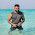can u suggest some other ways for question 5? i hav done another method but cant do same for question 5, 2nd

1.See the update blog page. Method II is added for 5(ii). Hope it helps!

2.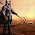This comment has been removed by the author.

2.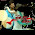i want to understand factorise questions.

3.its simple just do with the help of long division and after wise factorise it

4.wow! thanks a lot

5.Thanks a lot, You really make it easy for me and may be for everyone...............

6.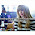I'm in eighth but i studied the topic outta curiousity . however I was stuck on a question . This thing really helped !

7.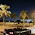where can i find the other method of Q5

8.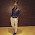thanku for making it easy

9.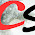thnx for making it easy..thanks a lot

10.Yeahh! It's very easy method
I understand very easily
Thank you very much🙏🙏